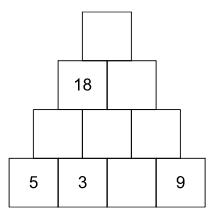# January 2023 - Problem of the Month

In the puzzle below, the value of a square in any of the top three levels is the sum of the two squares directly beneath it.  What will be the value in the top square?Solution

The top square will have a value of 44.  If the 5 in the bottom level is changed to a 6, what will the value of the top square?  If the 5 in the bottom level is not changed but the 3 in that level is changed to a 4, what will be the value of the top square?

Department